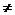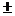2.12 Rational expressions of polynomials:

We have seen the following properties of polynomials:

If p(x) is a polynomial then:

1.   Sum/difference/Product of two polynomials is a polynomial

2.   p(x)+0 = p(x)

3.   p(x)-p(x) = 0

4.   p(x)*1 =p(x)

Thus, operations of polynomials are similar to the operations we carry out on integers.

Also if m, n and p are integers, we have learnt that (m/n)+(p/n) = (m+p)/n and (m/n)+(p/q) = (mq+np)/nq.

Integers and polynomials are algebraically similar.

An expression of the form p(x)/q(x) where p(x) and q(x) are polynomials and q(x)0 is called a ‘rational expression of polynomial’.

Note: A rational expression is always represented in its lowest term.(Numerator and denominator should not have any common factors. If they have, then divide them by their HCF).

Notes:

If p(x)/q(x) and g(x)/h(x) are two rational expressions, then:

1.   {p(x)/q(x)}*{g(x)/h(x)} = {p(x)*g(x)}/{q(x)*h(x)}

2.   {p(x)/q(x)}/{g(x)/h(x)} = {p(x)*h(x)}/{q(x)*g(x)}

3.   {p(x)/q(x)}{g(x)/h(x)} = {p(x)*h(x){q(x)*g(x)}/{q(x)*h(x)}

4.   p(x)/q(x) + r(x)/s(x) = {p(x)*s(x)+q(x)r(x)}/{q(x)s(x)}

1.   p(x)/q(x) - p(x)/q(x) = 0 (-p(x)/q(x) is called ‘additive inverse’ of p(x)/q(x))

2.   {p(x)/q(x)}/{p(x)/q(x)} = 1 (q(x)/p(x) is called ‘multiplicative inverse’ of p(x)/q(x))

2.12 Problem 1: What should be subtracted from (x2+2)/(x-1) to get (x-3)/(x+1) ?

Solution:

Let p(x)/q(x) be the rational expression to be subtracted(x2+2)/(x-1) - p(x)/q(x) = (x-3)/(x+1)(x2+2)/(x-1) - (x-3)/(x+1) =p(x)/q(x)

LHS = {(x2+2)(x+1)(x-1)(x-3)}/ {(x+1)(x-1)}

= {(x3+x2+2x+2) - (x2-3x-x+3)}/{(x2-1)}

= {(x3+x2+2x+2) - x2+4x-3}/{(x2-1)}

= {(x3+6x-1}/{(x2-1)}

Thus the rational expression to be subtracted is {(x3+6x-1}/{(x2-1)}

2.12  Problem 2. Simplify 1/(x+a) + 1/(x+b)+1/(x+c) +ax/(x3+ax2)  +bx/(x3+bx2) + cx/(x3+cx2)

Solution:

The given equation =

1/(x+a) + ax/(x3+ax2) +1/(x+b)+ bx/(x3+bx2) +1/(x+c) + cx/(x3+cx2)( Rearrange terms)

= {1/(x+a)} + {ax/x2(x+a)} + {1/(x+b)} + {bx/x2(x+b)} +{1/(x+c)} + {cx/x2(x+c)} (Take common factor out)

= {1/(x+a)} + {a/x(x+a)} + {1/(x+b)} + {b/x(x+b)} + {1/(x+c)} + {c/x(x+c)}( Cancel same terms)

= {(x+a)/x(x+a)} + {(x+b)/x(x+b)} + {(x+c)/x(x+c)} ( Simplify)

= 1/x+1/x+1/x = 3/x

2.12 Problem 3. If P = (x3+y3)/{(x-y)2+3xy}, Q = {(x+y)2-3xy}/ (x3-y3) and R = xy/(x2-y2)

Find (P/Q)*R

Solution:

P = (x3+y3)/ {(x-y)2+3xy}

= (x+y) (x2-xy+y2)/ {(x2-2xy+y2)+3xy)} (Apply formula for (x3+y3) and (x-y)2)

=(x+y) (x2-xy+y2)/ (x2+xy+y2)

Q = {(x+y)2-3xy}/ (x3-y3)

= {(x2+2xy+y2)-3xy)}/ {(x-y) (x2+xy+y2)} (Apply formula for (x3-y3) and (x+y)2)

= {(x2-xy+y2)}/ {(x-y) (x2+xy+y2)}

R = xy/(x2-y2)

=xy/{(x+y)(x-y)}P/Q = {(x+y) (x2-xy+y2)/ (x2+xy+y2)}/ [{(x2-xy+y2)} /{ (x-y) (x2+xy+y2)}]

= {(x+y) (x2-xy+y2)/ (x2+xy+y2)}*[{(x-y) (x2+xy+y2)}/ {(x2-xy+y2)}] (Reciprocal)

= (x+y)(x-y)P/Q*R = (x+y)(x-y) * {xy/{(x+y)(x-y)}

= xy

2.12 Summary of learning

 No Points to remember 1 Rules of mathematical operations (+,-,*, / ) on rational expressions of polynomials follow the same rules that are applicable to rational numbers